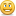# Feedback for a partial correct answers

Hello, Does Articulate Quizmaker allow for a partial correct feedback e.g. the user has given 3 correct mcq answers out of 5. Rather than 0 out of 5 or 5 out of 5?  I don't think it does, but just wanted to check as I'm being asked about this.

Thanks

###### 8 RepliesHi Susan! Quizmaker does offer a multiple response question type, but there's really no way to provide for partial scoring on this question type. The reason is that it would make authoring that type of question really difficult because the number of possible of choices. For example, say you have a question with just 3 choices (A,B,C)... so the possible answers would be:

A

A+B

A+B+C

A+C

B

B+C

C

So with only 3 questions, you have a possible combination of 7 responses. If you increased to FOUR choices A, B, C, D, the possible answers would be:

A

A+B

A+B+C

A+B+C+D

A+C

A+C+D

A+D

B

B+C

B+C+D

B+D

C

C+D

D

...and you can see how this would grow as the number of choices increase. Sometimes folks say, well how about if we just assign point values to A, B, C, so if the the correct answer is A+B, you could assign A - 5 points, B - 5 points, but that doesn't work because what happens if someone chooses A+B+C?  They would get the same score if they had chosen just A+B.

These are some of the reasons why we don't support partial scoring on multiple response. Hope that helps explain the logic.I read the rationale for why scoring this type of question is difficult but what if...

For a question with choices A, B, C and D- where let's say A B and C are correct - or should be selected - and D should not be selected. This would have to be a four point question (or multiple of 4) since 4 choices.

But the way points are assigned is whether or not you select the appropriate choices for each one individually.

So for example you get a point each if you select A, B or C and you also get a point if you do not select D. 4 points.

Works in all the other scenarios too.

It is almost like all four are individula T or F questions.

Make sense? Could Articulate program it that way?

I might be totally wrong based on capabilities of program but thought i would mention it....who knows.

ReginaRegina Lehotay

I read the rationale for why scoring this type of question is difficult but what if...

For a question with choices A, B, C and D- where let's say A B and C are correct - or should be selected - and D should not be selected. This would have to be a four point question (or multiple of 4) since 4 choices.

But the way points are assigned is whether or not you select the appropriate choices for each one individually.

So for example you get a point each if you select A, B or C and you also get a point if you do not select D. 4 points.

Works in all the other scenarios too.

It is almost like all four are individula T or F questions.

Make sense? Could Articulate program it that way?

I might be totally wrong based on capabilities of program but thought i would mention it....who knows.

Regina

I have exactly the same need for a quiz I'm creating - I see this was never responded to by Articulate. Any development on this?